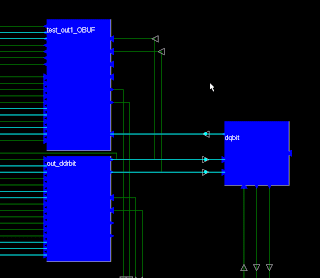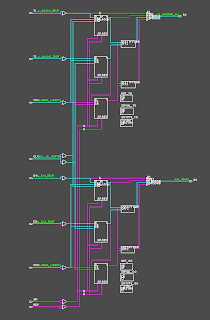## Monday, September 28, 2009

### OFFSET IN constraints on diff inputs ignored in IDS 11.3. Fixed in 12.4

Below is a simple test case with differential input clock and data.
````timescale 1ns / 1ps

module s3a_ibufds (
input  clk_i_p, clk_i_n,
input  d_i_p, d_i_n,
output d_o_p, d_o_n
);

wire d_in, clk_in;
reg d_r1, d_r2;

IBUFDS #(
.IBUF_DELAY_VALUE("0"),    // Specify the amount of added input delay for
//    the buffer: "0"-"16" (Spartan-3A)
.IFD_DELAY_VALUE("AUTO"),  // Specify the amount of added delay for input
//    register: "AUTO", "0"-"8" (Spartan-3A)
.IOSTANDARD("DEFAULT")     // Specify the input I/O standard
) IBUFDS_clk (
.O  (clk_in),  // Buffer output
.I  (clk_i_p),  // Diff_p buffer input (connect directly to top-level port)
.IB (clk_i_n) // Diff_n buffer input (connect directly to top-level port)
);

IBUFDS #(
.IBUF_DELAY_VALUE("0"),    // Specify the amount of added input delay for
//    the buffer: "0"-"16" (Spartan-3A)
.IFD_DELAY_VALUE("AUTO"),  // Specify the amount of added delay for input
//    register: "AUTO", "0"-"8" (Spartan-3A)
.IOSTANDARD("DEFAULT")     // Specify the input I/O standard
) IBUFDS_d (
.O  (d_in),  // Buffer output
.I  (d_i_p),  // Diff_p buffer input (connect directly to top-level port)
.IB (d_i_n) // Diff_n buffer input (connect directly to top-level port)
);

always @(posedge clk_in) begin
d_r1 <= d_in;
d_r2 <= d_r1;
end

assign d_o_p = d_r2;

endmodule

```
When the OFFSET IN constraints are specified with input nets,  they are simply ignored by the timing analyzer in ISE 11.3 (see UCF constraints and TA snapshot below):

```NET "clk_i_p" TNM_NET = clk_i_p;
TIMESPEC TS_clk_i_p = PERIOD "clk_i_p" 20 ns HIGH 50%;
NET "d_i_p" OFFSET = IN 2 ns VALID 4 ns BEFORE "clk_i_p" RISING;
NET "d_i_n" OFFSET = IN 2 ns VALID 4 ns BEFORE "clk_i_p" RISING;
```

This is probably caused by a bug in TA. As a workaround, the OFFSET IN constraints can be also specified with TIMEGRP and the tool will correctly analyze the timing constraint (see the UCF constraints and TA snapshot below).
[Update Feb 10th, 2011: Verified that the bug has been fixed in IDS 12.4] The project archive can be downloaded here in case anybody is interested.

```NET "clk_i_p" TNM_NET = clk_i_p;
TIMESPEC TS_clk_i_p = PERIOD "clk_i_p" 20 ns HIGH 50%; ```
```INST "d_i_p" TNM = TN_d_pads;
INST "d_i_n" TNM = TN_d_pads;
TIMEGRP "TN_d_pads" OFFSET = IN 2 ns VALID 4 ns BEFORE clk_i_p; ```
` `

## Wednesday, September 23, 2009

### Virtex6 Configuration Examples

The web links below will bring you directly to the block diagram for the selected configuration mode from the "Virtex6 FPGA Configuration User Guide" (UG360 v2.0 Nov 15, 2009). This is also the landing page for ADEPT (website and blog) when the "Example" button on the "Special Pin Setup" window is pressed.

## Friday, September 18, 2009

### Virtex4 ODDR tristate control

The tristate control of the tristate buffer in IOB is active low. It's important to code this in RTL for the tool to correctly implement it in HW. e.g.

assign dqbit = (ts_ddrbit == 1'b0) ? out_ddrbit : 1'bz;

A code example with IDDR, ODDRs for both the data and tristate control in Virtex4 is shown below. It's tested with Xilinx IDS 11.3.

``timescale 1ns / 1psmodule tristate_oddr(    input iddr_clk,    input oddr_clk,    inout dqbit,    input ts_ddrbit1,  input ts_ddrbit2,  input dq_bit1,  input dq_bit2,     output test_out1,  output test_out2    );wire out_ddrbit, ts_ddr_bit;ODDR #(    .DDR_CLK_EDGE("OPPOSITE_EDGE"), // "OPPOSITE_EDGE" or "SAME_EDGE"    .INIT   (1'b0), // Initial value of Q: 1’b0 or 1’b1    .SRTYPE ("SYNC") // Set/Reset type: "SYNC" or "ASYNC") oddr_out_ddrbit (    .Q  (out_ddrbit), // 1-bit DDR output    .C  (oddr_clk), // 1-bit clock input    .CE (1'b1), // 1-bit clock enable input    .D1 (dq_bit1), // 1-bit data input (positive edge)    .D2 (dq_bit2), // 1-bit data input (negative edge)    .R  (1'b0), // 1-bit reset    .S  (1'b0) // 1-bit set);ODDR #(    .DDR_CLK_EDGE("OPPOSITE_EDGE"), // "OPPOSITE_EDGE" or "SAME_EDGE"    .INIT   (1'b0), // Initial value of Q: 1’b0 or 1’b1    .SRTYPE ("SYNC") // Set/Reset type: "SYNC" or "ASYNC") oddr_ts_ddrbit (    .Q  (ts_ddrbit), // 1-bit DDR output    .C  (oddr_clk), // 1-bit clock input    .CE (1'b1), // 1-bit clock enable input    .D1 (ts_ddrbit1), // 1-bit data input (positive edge)    .D2 (ts_ddrbit2), // 1-bit data input (negative edge)    .R  (1'b0), // 1-bit reset    .S  (1'b0) // 1-bit set);//IMPORTANT: the tristate control of the tristate buffer in IOB is active low.assign dqbit = (ts_ddrbit == 1'b0) ? out_ddrbit : 1'bz;IDDR #(    .DDR_CLK_EDGE ("OPPOSITE_EDGE"), // "OPPOSITE_EDGE", "SAME_EDGE"    // or "SAME_EDGE_PIPELINED"    .INIT_Q1 (1'b0), // Initial value of Q1: 1’b0 or 1’b1    .INIT_Q2 (1'b0), // Initial value of Q2: 1’b0 or 1’b1    .SRTYPE  ("SYNC") // Set/Reset type: "SYNC" or "ASYNC") IDDR_dqbit (    .Q1 (test_out1), // 1-bit output for positive edge of clock    .Q2 (test_out2), // 1-bit output for negative edge of clock    .C  (iddr_clk), // 1-bit clock input    .CE (1'b1), // 1-bit clock enable input    .D  (dqbit), // 1-bit DDR data input    .R  (1'b0), // 1-bit reset    .S  (1'b0) // 1-bit set);endmodule`

Below is what the implementation looks like in FPGA_EDITOR:

IDDR, ODDR and IOBUF:Inside ODDR:Inside IOB: